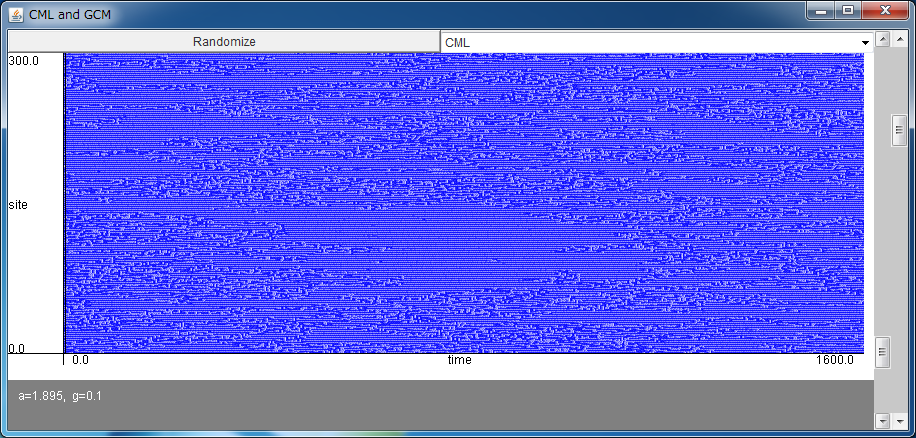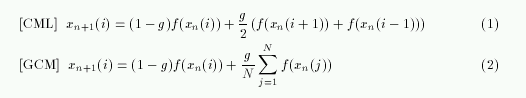# Coupled Map Lattice and Globally Coupled MapAfter downloading maplattice.jar, please execute it by double-clicking, or typing "java -jar maplattice.jar".

If the above application does not start, please install OpenJDK from adoptopenjdk.net.

CML (coupled map lattice) and GCM (globally coupled map) are the discrete-time map written byrespectively. CML couples the N chaotic maps xn+1=f(xn) locally, and GCM couples them globally.

As the chaotic map f(x), let us consider the logistic map f(x) = 1-a x2. Note that this map is equivalent to the well-known form g(z) = r z(1-z)
when the transformations a=r(r-2)/4 and x=2(2z-1)/(r-2) are taken.

These models were proposed by Dr. Kunihiko Kaneko, to use as general models for the complex high-dimensional dynamics, such as biological systems, networks of DNA, economic activities, neural networks, evolution, and so on.

The nonlinear dynamics and the chaos theory succeeded in understanding the low-dimensional complex dynamics where the number of variables which govern the system (e.g, 3 in Lorenz equation), or the number of positive Lyapunov exponents (e.g, 1 in Lorenz attractor), is small.
In such systems, the knowledge based on the analyses of the low-dimensional universal systems, e.g., logistic map, was efficiently utilized, but, it is insufficient for the analysis of the high-dimensional complex system, and a new recipe would be required.
The riddled basin, the on-off intermittency, the coupled map lattice (CML), and the globally coupled map (GCM) would be examples of them.

CML and GCM connect the many chaotic maps. To judge whether such a violent simplification is effective in modeling the nature, we should wait for the progress of the research on complex system. It is important that the chaotic itinerancy, which is often observed in many high-dimensional chaotic systems, was found in GCM.

This simulator shows the behavior of the model with 200-coupled logistic maps.
You can select CML or GCM with the upper choice, and you can change the values of the nonlinear parameter a and the coupling strength g with the right bars.
The output xn(i) of the site i is shown in every two time steps. The blue color means the large xn(i).

The following phenomena are typically observed.

[CML]
• frozen chaos: a<1.5
The sites are divided into the clusters with various sizes. The divided pattern can be regarded as an attractor.
With the different initial condition, the pattern of the clusters will be changed, thus it is known that the many attractors co-exist in the system.
The number of attractors increases (at least) exponentially with the increase of N.

• pattern selection: e.g., a=1.71, g=0.4
The sites are divided into the clusters with almost the same sizes. The size of the clusters depends on the parameters.

• chaotic Brownian motion of defect: e.g., a=1.85, g=0.1
In the case of the above pattern selection, some phase defects sometimes remain in the system.
These defects fluctuates chaotically like Brownian motion.

• defect turbulence: e.g., a=1.895, g=0.1
The many defects are generated and collide each other like the turbulence.

• spatiotemporal intermittency: e.g., a=1.75, g=0.3
The each site transits between the coherent state and the chaotic state intermittently.

• fully developed spatiotemporal chaos: e.g., a=2.0, g=0.3
Almost all the sites oscillate chaotically independently.

• traveling wave: e.g., a=1.47, g=0.5
The wave of clusters travels at the very low speed.

[GCM]
When the coupling strength g is increased, the transition
• asynchronous state -> partially synchronous state (ordered phase) -> synchronous state (coherent phase)
is observed. With the parameters with medium values, chaotic itinerancy is also observed (e.g., a=1.95, g=0.2).
This is a phenomenon where the synchronized clusters are sometimes rearranged chaotically, which is often observed in the high-dimensional dynamical system where many chaotic attractors co-exist. The chaotic pattern transitions in associative memory would be an example of the chaotic itinerancy.

In both CML and GCM, the many attractors co-exist in the high-dimensional phase space.

This page is based on the following textbook.